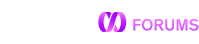GEOGRAPHY_WITHIN_DISTANCE with Point

Hi,

Below Query works fine. But, I want to use POINT instead, since I am having hard time to create geography_point using Spring JPA.

select * from house where
GEOGRAPHY_WITHIN_DISTANCE(geography_point(-96.92366, 32.83606), geography_point(lngtd_num,lattitude_num), 10);

Same Query using POINT is not working. I think it is not able to replace column values.
select * from house where
GEOGRAPHY_WITHIN_DISTANCE(“POINT(-96.92366 32.83606)”, “POINT(lngtd_num lattitude_num)”, 10);

Consider working around this issue by adding a computed column to your table that has the GEOGRAPHY type.

Example column definition

CREATE TABLE house (
...
location as geography_point(lngtd_num, lattitude_num) persisted geographypoint,
...
);

Then you rewrite the query as:

select * from house where
GEOGRAPHY_WITHIN_DISTANCE(“POINT(-96.92366 32.83606)”, location, 10);

Some of the relevant docs:

Hi, Thanks for the response.
I was able to figure a work around to use GEOGRAPHY_WITHIN_DISTANCE with two geography_point. Now, I have a question on how can I order these by distance, without having to use GEO function twice.

Below works fine, however GEO functions are used twice and was trying to favor GEOGRAPHY_WITHIN_DISTANCE instead, since it is faster as per the documentation.
select * from house where
GEOGRAPHY_WITHIN_DISTANCE(geography_point(-96.92366, 32.83606), geography_point(lngtd_num,lattitude_num), 10)
order by GEOGRAPHY_DISTANCE(geography_point(-96.926688, 32.83605), geography_point(lngtd_num, lattitude_num)) asc;

Your query seems appropriate. Given this query, you should consider adding an index on the location column. This might significantly reduce the runtime if your filter is very selective.

You can post the output of the following to find out what the query will do.

EXPLAIN select * from house where
GEOGRAPHY_WITHIN_DISTANCE(geography_point(-96.92366, 32.83606), geography_point(lngtd_num,lattitude_num), 10)
order by GEOGRAPHY_DISTANCE(geography_point(-96.926688, 32.83605), geography_point(lngtd_num, lattitude_num)) asc;